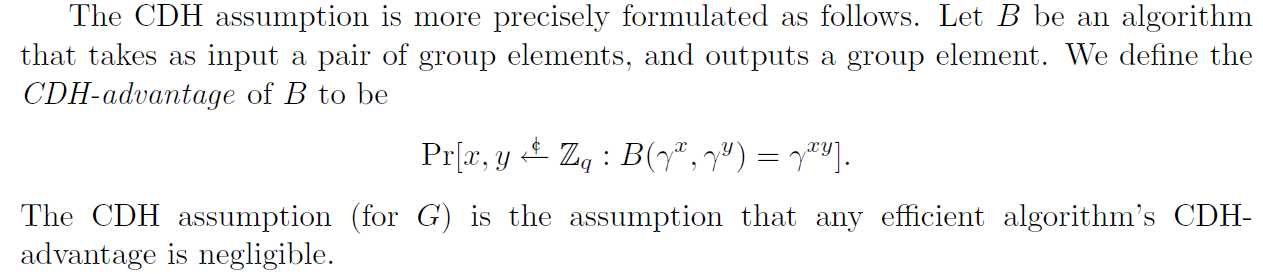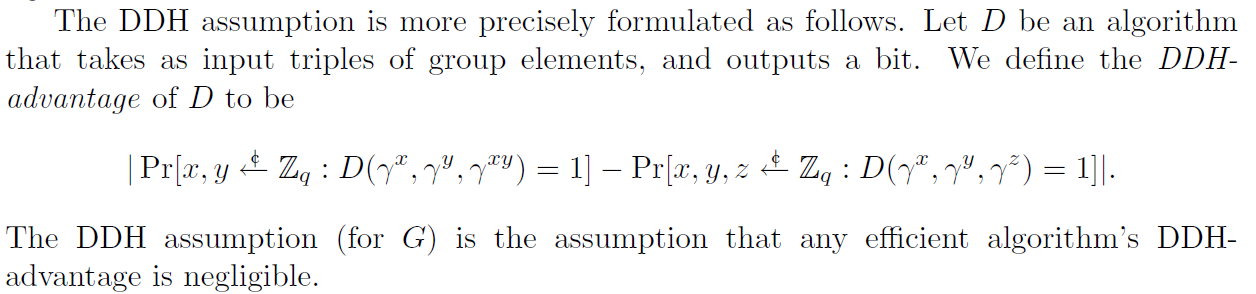Diffie-Hellman就是著名的DH密钥交换协议，用它做第一篇可证明安全学习笔记的原因有俩，一个是@Bintou在上周组会中刚好用DH做栗子给新人讲了可证明安全，另一个原因是，DH的协议比较简单，而且有一系列专门为它定制的难题，所以规约的过程也会相对简单

# 前置知识·

## 协议流程·

PS：一般$q$为素数，即$G$为素阶群

$\begin{array}{|l|} \hline \begin{array}{lcl} \text{P_i} & \ \ \ \ \ \ \ \ \ \ \ \ \ & \text{P_j} \\ \begin{array}{l} \\ x \overset{\}{\leftarrow} \mathbb{Z}_{q} \\ X = g^x \text{ (in G)} \end{array} & & \begin{array}{l} \\ y \overset{\}{\leftarrow} \mathbb{Z}_{q} \\ Y = g^y \text{ (in G)} \end{array}\\ & \overset{X}{\longrightarrow} & \\ & \overset{Y}{\longleftarrow} & \\ \begin{array}{l} K_i = Y^x \text{ (in G)} \\ \end{array} & & \begin{array}{l} K_j = X^y \text{ (in G)} \\ \end{array}\\ \end{array} \\ \hline \end{array}$

$K_i = Y^x = g^{yx} = g^{xy} = X^y = K_j \text{ (in G)}$

## 可证明安全·

• 安全定义：即定义一个协议符合什么条件才是安全的，通常在安全定义中会界定攻击者的攻击能力，即攻击者只能进行什么攻击，不能进行什么攻击
• 难题：顾名思义，就是一些被证明的或者公认的多项式时间内不可解决的问题，关于难题可以去复习一下计算理论
• 规约：比如说把问题$A$规约到问题$B$，大概的意思是，如果问题$B$被解了，那么问题$A$也可被解，关于规约也可以去复习一下计算理论

• 首先构造一种规约，把某个难题规约到安全定义，详细点说即，如果存在一个符合我安全定义的攻击能力的攻击者，可以攻破我安全定义中设定的条件的话，那么就可以使用这个攻击者去解决这个难题
• 规约完成后，即是如果安全定义被攻击则难题被攻击，$\text{安全定义} \to \text{难题}$，如果把这个命题逆否一下，即$\neg\text{难题} \to \neg\text{安全定义}$，根据假设，难题在多项式时间内不可解，所以安全定义也在多项式时间内不可被攻破
• 另外，详细来说，在以上规约中，还需要一个模拟者$\text{S}$来模拟规约的过程，即假设存在一个攻击者$\text{A}$能够攻破我的安全定义，那么模拟者则需要通过调用$\text{A}$来解决难题

PS：多嘴说一下，以上说的规约定义都是按照计算理论抄的，在别的论文或文章中会有一种像“方案的安全性可以规约到xxx问题”的说法，这里的“规约”比较像是这个方案基于xxx问题的意思，注意区分就好

## 安全难题·

### CDH假设·$G$为循环群，$g$为其生成元，$q$为其群阶，在$\mathbb{Z}_q$中均匀随机选择$x$$y$，若存在攻击者$\text{A}$，在仅知道公开信息$(G, g, q)$和输入$(g^x, g^y)$的情况下，可以在多项式时间内计算出$g^{xy}$，则称攻击者$\text{A}$攻破了CDH难题

$\text{Pr} \begin{bmatrix} \begin{array}{ccc} \text{A}(g^x, g^y) = g^{xy} & \text{:} & x, y \overset{\}{\leftarrow} \mathbb{Z}_{q} \end{array} \end{bmatrix}$

$\text{Adv}_\text{CDH}(\text{A}) := \text{Pr} \begin{bmatrix} \begin{array}{ccc} \text{A}(g^x, g^y) = g^{xy} & \text{:} & x, y \overset{\}{\leftarrow} \mathbb{Z}_{q} \end{array} \end{bmatrix}$

PS：个人认为准确点说应该是

$\text{Adv}_\text{CDH}(\text{A}) := \begin{vmatrix} \text{Pr} \begin{bmatrix} \begin{array}{ccc} \text{A}(g^x, g^y) = g^{xy} & \text{:} & x, y \overset{\}{\leftarrow} \mathbb{Z}_{q} \end{array} \end{bmatrix} - \frac{1}{q} \end{vmatrix}$

CDH假设声称CDH问题不可被解决，则不存在优势不可忽略的多项式攻击者

### DDH假设·$G$为循环群，$g$为其生成元，$q$为其群阶，在$\mathbb{Z}_q$中均匀随机选择$x$$y$$z$，若存在攻击者$\text{A}$，在仅知道公开信息$(G, g, q)$和输入$(g^x, g^y, Z)$的情况下，可以分辨$Z = g^{xy}$还是$Z = g^z$，则称攻击者$\text{A}$攻破了DDH难题

\text{Adv}_\text{DDH}(\text{A}) := \begin{vmatrix} \text{Pr} \begin{bmatrix} \begin{array}{ccc} \text{A}(g^x, g^y, Z) = b & \text{:} & \begin{array}{l} x, y, z \overset{\}{\leftarrow} \mathbb{Z}_{q} \text{; } b \overset{\}{\leftarrow} \{0, 1\} \\ Z = \left\{\begin{aligned} g^{xy} &, b = 0 \\ g^{z} &, b = 1 \end{aligned}\right. \end{array} \end{array} \end{bmatrix} - \frac{1}{2} \end{vmatrix}

$\text{Adv}_\text{DDH}(\text{A}) := \begin{vmatrix} \text{Pr} \begin{bmatrix} \begin{array}{ccc} \text{A}(g^x, g^y, g^{xy}) = 1 & \text{:} & x, y \overset{\}{\leftarrow} \mathbb{Z}_{q} \end{array} \end{bmatrix} - \text{Pr} \begin{bmatrix} \begin{array}{ccc} \text{A}(g^x, g^y, g^{z}) = 1 & \text{:} & x, y, z \overset{\}{\leftarrow} \mathbb{Z}_{q} \end{array} \end{bmatrix} \end{vmatrix}$

DDH假设声称DDH问题不可被解决，则不存在优势不可忽略的多项式攻击者

### DDH规约到CDH·

• 在接收到$(g^x, g^y, Z)$后，把$(g^x, g^y)$输入给攻击者$\text{A}$
• 接收到攻击者$\text{A}$输出的$Z_A$后，对比$Z_A$$Z$是否相等，若相等则输出$0$，否则输出$1$

$\text{Adv}_\text{DDH}(\text{S}^\text{A}) \ge \text{Adv}_\text{CDH}(\text{A})$

# 01-最简单的栗子（CDH）·

$\begin{array}{|l|} \hline \begin{array}{lcl} \mathrm{P_i} & \ \ \ \ \ \ \ \ \ \ \ \ \ & \mathrm{P_j} \\ \begin{array}{l} \\ x \overset{\}{\leftarrow} \mathbb{Z}_{q} \\ X = g^x \text{ (in G)} \end{array} & & \begin{array}{l} \\ y \overset{\}{\leftarrow} \mathbb{Z}_{q} \\ Y = g^y \text{ (in G)} \end{array}\\ & \overset{X}{\longrightarrow} & \\ & \overset{Y}{\longleftarrow} & \\ \begin{array}{l} K_i = Y^x \text{ (in G)} \\ \end{array} & & \begin{array}{l} K_j = X^y \text{ (in G)} \\ \end{array}\\ \end{array} \\ \hline \end{array}$

$\begin{array}{|ll|} \hline & \text{DH(G, g, q):} \\ \textcolor{lightgray}{01} & x, y \overset{\}{\leftarrow} \mathbb{Z}_{q} \\ \textcolor{lightgray}{02} & X = g^x \text{ (in G)} \\ \textcolor{lightgray}{03} & Y = g^y \text{ (in G)} \\ \textcolor{lightgray}{04} & K = g^{xy} \text{ (in G)} \\ \textcolor{lightgray}{05} & \text{return X, Y, K} \\ \hline \end{array}$

$\text{Adv}_\text{DH01}(\text{A}) := \text{Pr} \begin{bmatrix} \begin{array}{ccc} \text{A}(X, Y) = K & \text{:} & \begin{array}{l} G, g, q \leftarrow \text{Gen()} \\ X, Y, K \leftarrow \text{DH(G, g, q)} \end{array} \end{array} \end{bmatrix}$

1. 在接收到CDH问题输入的$(g^x, g^y)$后，给$\text{A}$输入$(g^x, g^y)$

2. 接收到$\text{A}$输出的$K$后，输出$K$

$\text{Adv}_\text{CDH}(\text{S}^\text{A}) \ge \text{Adv}_\text{DH01}(\text{A}) > \text{negl}$

# 02-猜比特（DDH）·

$\text{Adv}_\text{DH02}(\text{A}) := \begin{vmatrix} \text{Pr} \begin{bmatrix} \begin{array}{ccc} \text{A}(X, Y, K_b) = b & \text{:} & \begin{array}{l} G, g, q \leftarrow \text{Gen()} \\ X, Y, K_0 \leftarrow \text{DH(G, g, q)} \\ K_1 \overset{\}{\leftarrow} G \text{; } b \overset{\}{\leftarrow} \{0, 1\} \\ \end{array} \end{array} \end{bmatrix} - \frac{1}{2} \end{vmatrix}$

1. 接收到DDH问题输入的$(g^x, g^y, Z)$后，把$(g^x, g^y, Z)$输入到$\text{A}$
2. 接收到$\text{A}$输出的$b$后，输出$b$

$\text{Adv}_\text{DDH}(\text{S}^\text{A}) \ge \text{Adv}_\text{DH02}(\text{A}) > \text{negl}$

# 03-多个Session（CDH）·

Session就是会话的意思，可以把DH协议中的一次密钥交换看作一个Session，即把上面协议图完整执行一遍后就是完成一个Session

（注：由于一时间没找到相关定义，以下定义的一些部分是我自己编的）

$\begin{array}{|ll|} \hline & \text{Init(n_s, G, g, q):} \\ \textcolor{lightgray}{01} & \mathcal{T} = [\ ] \\ \textcolor{lightgray}{02} & \mathcal{K} = [\ ] \\ \textcolor{lightgray}{04} & \text{for i from 0 to n_s-1:} \\ \textcolor{lightgray}{05} & \quad X_i, Y_i, K_i \leftarrow \text{DH(G, g, q)} \\ \textcolor{lightgray}{06} & \quad \mathcal{T}\text{.append((X_i, Y_i))} \\ \textcolor{lightgray}{07} & \quad \mathcal{K}\text{.append(K_i)} \\ \textcolor{lightgray}{08} & \text{return \mathcal{T}, \mathcal{K}} \\ \hline \end{array}$

$\text{Adv}_\text{DH03}(\text{A}) := \text{Pr} \begin{bmatrix} \begin{array}{ccc} \begin{array}{l} \text{A}(\mathcal{T}) \to K \\ K \in \mathcal{K} \end{array} & \text{:} & \begin{array}{l} G, g, q \leftarrow \text{Gen()} \\ \mathcal{T}, \mathcal{K} \leftarrow \text{Init(n_s, G, g, q)} \end{array} \end{array} \end{bmatrix}$

1. 在接收到CDH问题输入的$(g^x, g^y)$后，运行$\text{Init}(n_s-1)$获得$\mathcal{T}_\text{S}$$\mathcal{K}_\text{S}$，把$(g^x, g^y)$嵌入到$\mathcal{T}_\text{S}$随机位置，然后把$\mathcal{T}_\text{S}$输入给$\text{A}$
2. 接收$\text{A}$的输出$K$，然后输出$K$

$\text{Adv}_\text{CDH}(\text{S}^\text{A}) \ge \frac{1}{n_s} \text{Adv}_\text{DH03}(\text{A}) > \text{negl}$

## 更紧致的规约？·

（注意：以下只是个人观点，不一定对，）

1. 接收CDH问题输入的$(g^x, g^y)$
2. 运行$\text{Init}(n_s-1)$获得$\mathcal{T}_\text{S}$$\mathcal{K}_\text{S}$，把$(g^x, g^y)$嵌入到$\mathcal{T}_\text{S}$随机位置，然后把$\mathcal{T}_\text{S}$输入给$\text{A}$
3. 接收$\text{A}$的输出$K$，若$K \in \mathcal{K}_\text{S}$，则跳转到操作2，否则跳转到操作4
4. 输出$K$

$\text{Adv}_\text{CDH}(\text{S}^\text{A}) \ge \frac{\frac{1}{n_s} \text{Adv}_\text{DH03}(\text{A})} {1 - \text{Adv}_\text{DH03}(\text{A}) + \frac{1}{n_s} \text{Adv}_\text{DH03}(\text{A})} = \frac{\text{Adv}_\text{DH03}(\text{A})} {n_s - (n_s-1) \text{Adv}_\text{DH03}(\text{A})} > \text{negl}$

$\text{Adv}_\text{CDH}(\text{S}^\text{A}) \ge \frac{1}{n'} \text{Adv}_\text{DH03}(\text{A}) > \text{negl}$

# 04-多Session猜比特（CDH）·

$\begin{array}{|ll|} \hline & \text{Rand(n_s, G):} \\ \textcolor{lightgray}{01} & \mathcal{K} = [\ ] \\ \textcolor{lightgray}{02} & \text{for i from 0 to n_s-1:} \\ \textcolor{lightgray}{03} & \quad K_i \overset{\}{\leftarrow} G \\ \textcolor{lightgray}{04} & \quad \mathcal{K}\text{.append(K_i)} \\ \textcolor{lightgray}{05} & \text{return \mathcal{K}} \\ \hline \end{array}$

$\text{Adv}_\text{DH04}(\text{A}) := \begin{vmatrix} \text{Pr} \begin{bmatrix} \begin{array}{ccc} \text{A}(\mathcal{T}, \mathcal{K}_b) = b & \text{:} & \begin{array}{l} G, g, q \leftarrow \text{Gen()} \\ \mathcal{T}, \mathcal{K}_0 \leftarrow \text{Init(n_s, G, g, q)} \\ \mathcal{K}_1 \leftarrow \text{Rand(n_s, G)} \\ b \overset{\}{\leftarrow} \{0, 1\} \\ \end{array} \end{array} \end{bmatrix} - \frac{1}{2} \end{vmatrix}$

$\begin{array}{|l|} \hline \begin{array}{lcl} \mathrm{P_i} & \ \ \ \ \ \ \ \ \ \ \ \ \ & \mathrm{P_j} \\ \begin{array}{l} \\ x \overset{\}{\leftarrow} \mathbb{Z}_{q} \\ X = g^x \text{ (in G)} \end{array} & & \begin{array}{l} \\ y \overset{\}{\leftarrow} \mathbb{Z}_{q} \\ Y = g^y \text{ (in G)} \end{array}\\ & \overset{X}{\longrightarrow} & \\ & \overset{Y}{\longleftarrow} & \\ \begin{array}{l} K_i = \text{H}(Y^x \text{ (in G)}) \\ \end{array} & & \begin{array}{l} K_j = \text{H}(X^y \text{ (in G)}) \\ \end{array}\\ \end{array} \\ \hline \end{array}$

$\begin{array}{|ll|} \hline & \text{Init: } \mathcal{H} = [\ ] \\ \hline & \text{H(a):} \\ \textcolor{lightgray}{02} & \text{if a \not\in G: \bot } \\ \textcolor{lightgray}{03} & \text{if (a, *) \in G:} \\ \textcolor{lightgray}{04} & \quad (a, h_a) \leftarrow \mathcal{H} \\ \textcolor{lightgray}{04} & \quad \text{return h_a} \\ \textcolor{lightgray}{04} & h_a \overset{\}{\leftarrow} \{0, 1\}^\lambda \\ \textcolor{lightgray}{04} & \mathcal{H}\text{.append((a, h_a))} \\ \textcolor{lightgray}{05} & \text{return h_a} \\ \hline \end{array}$

1. （施工中）